# Embedding Matplotlib Animations in Jupyter as Interactive JavaScript Widgets

A while back I wrote a post on Embedding Matplotlib Animations in Jupyter Noteboks, which became surprisingly popular. It outlined how to render Matplotlib animations in the Jupyter Notebook, by encoding it as a HTML5 video using the to_html5_video method introduced in the release of Matplotlib 1.5.

Three years have gone by since then, and much has changed in the world of open-source scientific Python. Matplotlib 2.1 was released towards the end of last year, and was the first release with major new features since 1.5. Among many significant enhancements, the release included the merge of Jake Vanderplas' JSAnimation package, a tool for rendering Matplotlib animations as interactive JavaScript widgets.

With this awesome addition, encapsulated in the new to_jshtml method, we are now able to embed an interactive JavaScript widget for playback of Matplotlib animations directly inside a Jupyter Notebook.

In this post, we demonstrate how to use this method on the same example animation from my previous post, which was originally derived from an even earlier post by Jake VanderPlas.

# A Simple Illustration of Density Ratio Estimation and KL Divergence Estimation by Probabilistic Classification

##### Preamble¶
In :
%matplotlib notebook

In :
import tensorflow as tf
import numpy as np
import pandas as pd

import matplotlib.pyplot as plt
import seaborn as sns

from scipy.stats import norm
from scipy.special import expit, logit

from keras import backend as K
from keras.layers import Activation, Dense
from keras.models import Sequential, Model
from keras.losses import binary_crossentropy

from sklearn.model_selection import train_test_split

from keras.utils.vis_utils import model_to_dot, plot_model
from IPython.display import SVG

Using TensorFlow backend.

##### Notebook Environment¶
In :
plt.style.use('seaborn-notebook')
sns.set_context('notebook')
np.set_printoptions(precision=2,
edgeitems=3,
linewidth=80,
suppress=True)

In :
'TensorFlow version: ' + K.tf.__version__

Out:
'TensorFlow version: 1.4.1'
In :
sess = tf.InteractiveSession()

###### Constants¶
In :
seed = 8888
rng = np.random.RandomState(seed)

In :
golden_size = lambda width: (width, 2. * width / (1 + np.sqrt(5)))


## Simple 1D Gaussians Illustration¶

Consider the following 1D Gaussian distributions,

\begin{align} p(x) &= \mathcal{N}(x \mid 1, 1^2), \\ q(x) &= \mathcal{N}(x \mid 0, 2^2). \end{align}

In :
mu_p = 1.
sigma_p = 1.

In :
mu_q = 0.
sigma_q = 2.

In :
p = tf.distributions.Normal(loc=mu_p, scale=sigma_p)
q = tf.distributions.Normal(loc=mu_q, scale=sigma_q)

In :
xs = np.float32(np.linspace(-5., 5., 500))

In :
fig, ax = plt.subplots(figsize=golden_size(6))

ax.set_title('probability densities')

ax.plot(xs, q.prob(xs).eval(), label='$q(x)$')
ax.plot(xs, p.prob(xs).eval(), label='$p(x)$')

ax.set_xlim(-5.5, 5.5)

ax.set_xlabel('$x$')
ax.set_ylabel('density')

ax.legend()

plt.show()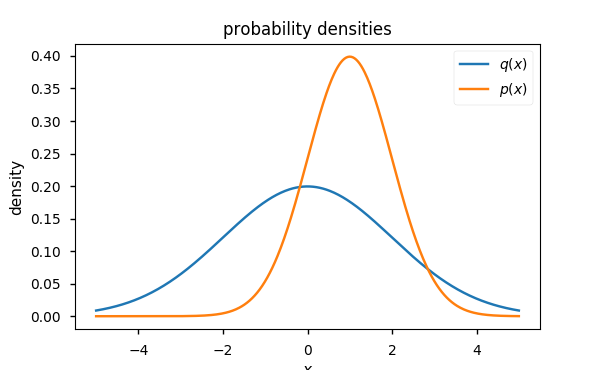### Density Ratio¶

The ratio of their probability densities is given by,

$$r(x) = \frac{p(x)}{q(x)}.$$

In :
density_ratio = lambda x, p, q: tf.truediv(p.prob(x), q.prob(x))

In :
density_ratios = density_ratio(xs, p, q).eval()

In :
fig, ax = plt.subplots(figsize=golden_size(6))

ax.set_title('density ratio')

ax.plot(xs, density_ratios)

ax.set_xlim(-5.5, 5.5)
ax.set_xlabel('$x$')

ax.set_ylabel('$r(x)$')

plt.show()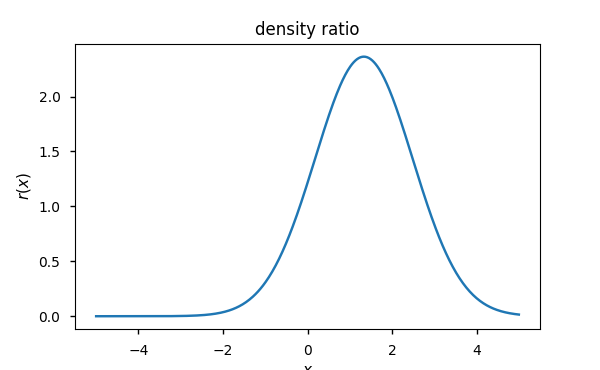#### Log density ratio of Gaussian distributions¶

The log of the density ratio between Gaussians can be simplied nicely as,

$$\log \sigma_q - \log \sigma_p + \frac{1}{2} \left \{ \left ( \frac{x-\mu_p}{\sigma_p}^2 \right ) - \left ( \frac{x-\mu_q}{\sigma_q}^2 \right ) \right \}.$$

In :
def log_density_ratio_gaussians(x, mu_p, sigma_p, mu_q, sigma_q):

r_p = (x - mu_p) / sigma_p
r_q = (x - mu_q) / sigma_q

return np.log(sigma_q) - np.log(sigma_p) + .5 * (r_q**2 - r_p**2)

In :
log_density_ratios = log_density_ratio_gaussians(
xs, mu_p, sigma_p, mu_q, sigma_q
)


We can verify this is correct,

In :
assert np.allclose(log_density_ratios, np.log(density_ratios))

In :
fig, ax = plt.subplots(figsize=golden_size(6))

ax.set_title('log density ratio')

ax.plot(xs, log_density_ratios)

ax.set_xlim(-5.5, 5.5)
ax.set_xlabel('$x$')

ax.set_ylabel('$r(x)$')

plt.show()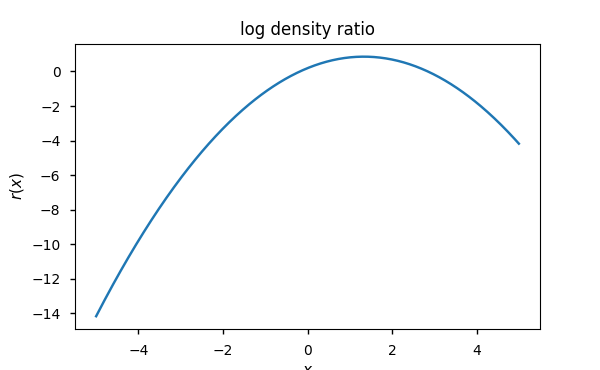### Density ratio estimation by probabilistic classification¶

Suppose now that we don't have access to the densities $p(x)$ and $q(x)$ for whatever reason, which is quite a common scenario. Density ratio estimation is concerned with directly estimating $r(x)$ using only samples from these distributions. Preferably, we can do this without resorting to estimating the individual densities $p(x)$ or $q(x)$, since the error in estimating the denominator $q(x)$ is magnified dramatically. One of the most simple approaches is probabilistic classification.

Let $\mathcal{D}_p = \{x_p^{(i)}\}_{i=1}^{n_p}$ and $\mathcal{D}_q = \{x_q^{(j)}\}_{j=1}^{n_q}$ be sets of samples drawn from distributions $p(x)$ and $q(x)$, respectively.

In :
n_p = n_q = 500

In :
samples_p = p.sample(sample_shape=(n_p,)).eval()
samples_q = q.sample(sample_shape=(n_q,)).eval()


We assign labels $y=1$ to samples from $\mathcal{D}_p$ and $y=0$ to samples from $\mathcal{D}_q$. Now the two densities can be rewritten as:

\begin{align} p(x) & = p(x \mid y = 1), \\ q(x) & = p(x \mid y = 0). \\ \end{align}

(Need to revise notation here... We're overloading $p$)

Let $n = n_p + n_q$, we form the dataset $\{ (x_k, y_k) \}_{k=1}^n$, where

\begin{align} (x_1, \dotsc, x_n) &= (x_p^{(1)}, \dotsc, x_p^{(n_p)}, x_q^{(1)}, \dotsc, x_q^{(n_q)}), \\ (y_1, \dotsc, y_n) &= (\underbrace{1, \dotsc, 1}_{n_p}, \underbrace{0, \dotsc, 0}_{n_q}). \end{align}

In :
x = np.hstack([samples_p, samples_q])
y = np.hstack([np.ones_like(samples_p),
np.zeros_like(samples_q)])

In :
x_train, x_test, y_train, y_test = train_test_split(
x, y, test_size=.2, random_state=rng
)

In :
fig, ax = plt.subplots(figsize=golden_size(6))

ax.scatter(x_test, y_test, c=y_test, s=10.**2,
marker='s', alpha=.2, cmap='coolwarm_r')

ax.set_xlim(-5.5, 5.5)

ax.set_xlabel('samples')
ax.set_yticks([0, 1])
ax.set_yticklabels(['$q(x)$', '$p(x)$'])

plt.show()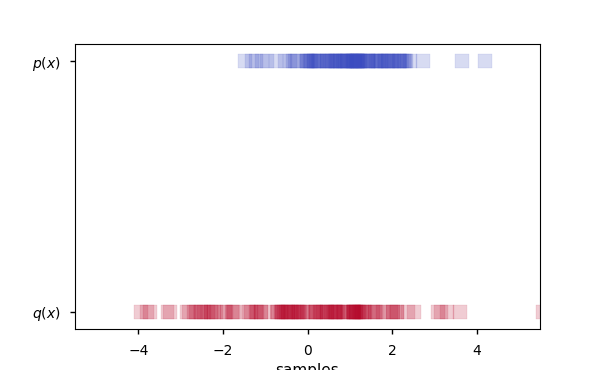Now, by Bayes's rule,

$$p(x \mid y) = \frac{p(y \mid x) p(x)}{p(y)}.$$

Hence, we can write the density ratio $r(x)$ in terms of class probabilities,

\begin{align} r(x) & = \frac{p(x)}{q(x)} \\ & = \frac{p(x \mid y = 1)}{p(x \mid y = 0)} \\ & = \left ( \frac{p(y = 1 \mid x) p(x)}{p(y = 1)} \right ) \left ( \frac{p(y = 0 \mid x) p(x)}{p(y = 0)} \right ) ^ {-1} \\ & = \frac{p(y = 0)}{p(y = 1)} \frac{p(y = 1 \mid x)}{p(y = 0 \mid x)} \end{align}

We can approximate the ratio of prior densities by the ratio of sample sizes:

$$\frac{p(y = 0)}{p(y = 1)} \approx \frac{n_q}{n_p + n_q} \frac{n_p + n_q}{n_p} = \frac{n_q}{n_p}$$

The class-posterior probability $p(y \mid x)$ is estimated by training a probabilitic classifier to discriminate between samples from $\mathcal{D}_p$ and $\mathcal{D}_q$. Then, an estimator of the density ratio $\hat{r}(x)$ can be constructed from an estimator of the class-posterior probability $\hat{p}(y \mid x)$,

$$\hat{r}(x) = \frac{n_q}{n_p} \frac{\hat{p}(y = 1 \mid x)} {\hat{p}(y = 0 \mid x)} = \frac{n_q}{n_p} \frac{\hat{p}(y = 1 \mid x)} {1 - \hat{p}(y = 1 \mid x)}.$$

To reduce clutter, let us assume for now that $n_p = n_q$ and denote the probabilistic classifier $D_{\theta}(x) := \hat{p}(y = 1 \mid x)$. Then we can write $\hat{r}(x)$ as

\begin{align} \hat{r}(x) = \frac{D(x)}{1 - D(x)} & = \exp \left ( \log \frac{D(x)}{1 - D(x)} \right ) \\ & = \exp \left ( \sigma^{-1}(D(x)) \right ). \\ \end{align}

#### Bayes optimal classifier¶

Conversely, the Bayes optimal classifier can be written as a function of the density ratio,

$$p(y=1 \mid x) = \sigma(\log r(x)) = \frac{p(x)}{p(x) + q(x)}.$$

In :
classifier_optimal = lambda x, p, q: tf.truediv(p.prob(x),
p.prob(x) + q.prob(x))

In :
y_pred_optimal = classifier_optimal(x_test, p, q).eval()

In :
fig, ax = plt.subplots(figsize=golden_size(6))

ax.set_title('Optimal class probabilities')

ax.scatter(x_test, y_test, c=y_test, s=10.**2,
marker='s', alpha=.2, cmap='coolwarm_r')

ax.scatter(x_test, y_pred_optimal, c=y_pred_optimal, cmap='coolwarm_r')

ax.set_xlim(-5.5, 5.5)
ax.set_xlabel('samples')

ax.set_yticks([0., .5, 1.])
ax.set_ylabel('$p(y=1|x)$')

plt.show()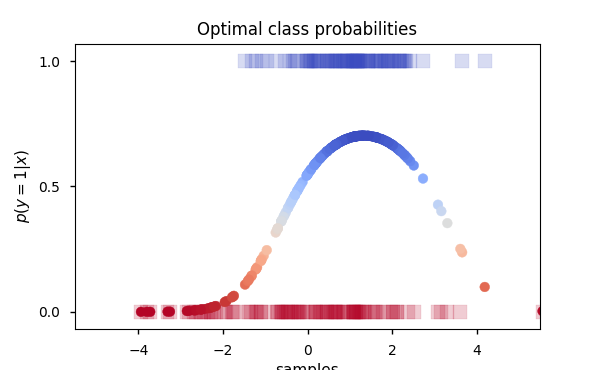#### Logistic regression¶

Perhaps the most conceptually straightforward approach for probabilistic classification is logistic regression.

We define a fully-connected neural network with two hidden layers and softplus activations.

In :
classifier = Sequential([
Dense(10, input_dim=1, activation='softplus'),
Dense(20, activation='softplus'),
Dense(1, name='log_odds'),
Activation('sigmoid')
])

In :
classifier.compile(optimizer='rmsprop', loss='binary_crossentropy')

In :
SVG(model_to_dot(classifier, show_layer_names=False, show_shapes=True)
.create(prog='dot', format='svg'))

Out:
In :
hist = classifier.fit(x_train, y_train,
shuffle=True,
batch_size=100,
epochs=250,
validation_data=(x_test, y_test),
verbose=0)

In :
fig, ax = plt.subplots(figsize=golden_size(6))

hist_df = pd.DataFrame(hist.history)
hist_df.plot(ax=ax)

ax.set_ylabel('loss')
ax.set_xlabel('epochs')

plt.show()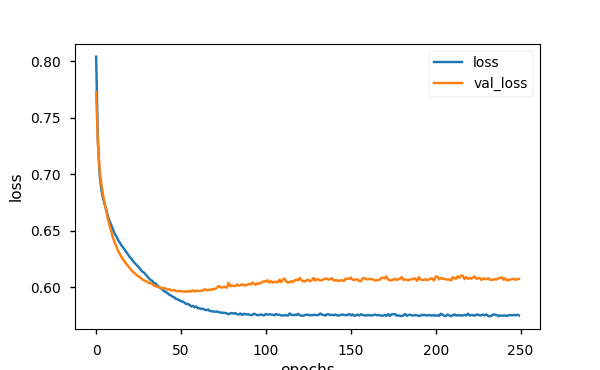In :
y_pred = classifier.predict(x_test).ravel()

In :
fig, ax = plt.subplots(figsize=golden_size(6))

ax.set_title('Estimated class probabilities')

ax.scatter(x_test, y_test, c=y_test, s=10.**2,
marker='s', alpha=.2, cmap='coolwarm_r')

ax.scatter(x_test, y_pred, c=y_pred, cmap='coolwarm_r')

ax.set_xlim(-5.5, 5.5)
ax.set_xlabel('$x$')

ax.set_ylabel('$D(x)$')

plt.show()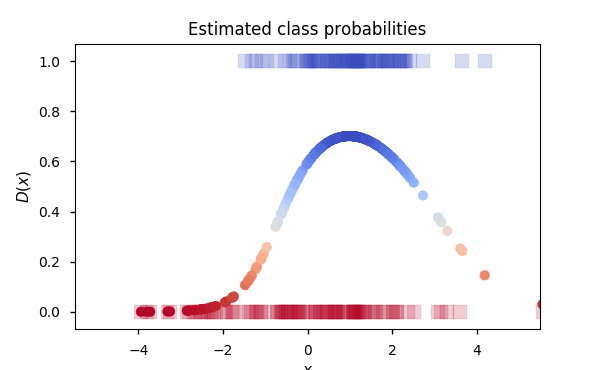In :
ys_optimal = classifier_optimal(xs, p, q).eval()
ys = classifier.predict(xs).ravel()

In :
fig, ax = plt.subplots(figsize=golden_size(6))

ax.set_title('Class probabilities')

ax.plot(xs, ys_optimal, label='Optimal')
ax.plot(xs, ys, label='Estimated')

ax.set_xlim(-5.5, 5.5)
ax.set_xlabel('$x$')

ax.set_ylim(0., 1.)
ax.set_ylabel('$p(y=1|x)$')

ax.legend()

plt.show()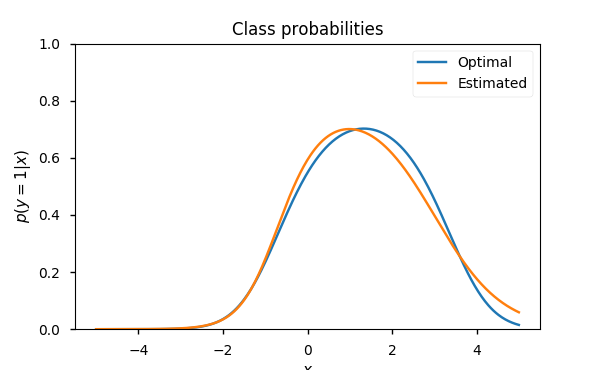#### Density ratio estimator¶

In :
log_ratio_estimator = Model(
classifier.input,
classifier.get_layer('log_odds').output
)

In :
log_odds = log_ratio_estimator.predict(xs).ravel()
log_density_ratios = log_density_ratio_gaussians(
xs, mu_p, sigma_p, mu_q, sigma_q
)

In :
assert np.allclose(ys, expit(log_odds))

In :
# side note: the following is true analytically
# but evaluates to false, even with some tolerance
np.allclose(xs, logit(expit(xs)))

Out:
False
In :
# hence this assert will actually fail but not
# for the reasons we actually care about
# assert np.allclose(logit(ys), log_odds)

In :
fig, ax = plt.subplots(figsize=golden_size(6))

ax.set_title('Log density ratio')

ax.plot(xs, log_density_ratios, label='Analytical')
ax.plot(xs, log_odds, label='Estimated')

ax.set_xlim(-5.5, 5.5)
ax.set_xlabel('$x$')

ax.set_ylabel('$\log r(x)$')

ax.legend()

plt.show()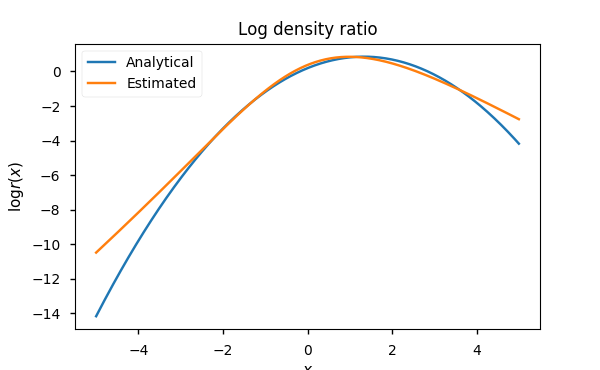In :
fig, ax = plt.subplots(figsize=golden_size(6))

ax.set_title('Density ratio')

ax.plot(xs, density_ratio(xs, p, q).eval(), label='Analytical')
ax.plot(xs, np.exp(log_odds), label='Estimated')

ax.set_xlim(-5.5, 5.5)
ax.set_xlabel('$x$')

ax.set_ylabel('$r(x)$')

ax.legend()

plt.show()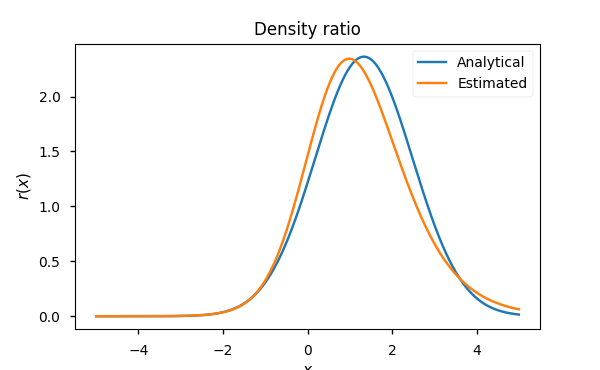### KL divergence estimation¶

A natural application of density ratio estimation is divergence estimation. Namely, approximating a divergence from the general family of $f$-divergences using only samples. Here, we will approximate the Kullback-Liebler (KL) divergence between $P$ and $Q$ without using their respective densities, $p(x)$ and $q(x)$.

#### Analytical¶

In :
tf.distributions.kl_divergence(p, q).eval()

Out:
0.44314718
##### KL divergence between Gaussians¶

For Gaussian distributions, the KL divergence can be evaluated analytically as,

$$\log \sigma_q - \log \sigma_p - \frac{1}{2} \left \{ 1 - \left (\frac{\sigma_p^2 + (\mu_p - \mu_q)^2}{\sigma_q^2} \right ) \right \}.$$

In :
def kl_divergence_gaussians(mu_p, sigma_p, mu_q, sigma_q):

r = mu_p - mu_q

return (np.log(sigma_q) - np.log(sigma_p)
- .5 * (1. - (sigma_p**2 + r**2) / sigma_q**2))

In :
kl_analytical = kl_divergence_gaussians(mu_p, sigma_p, mu_q, sigma_q)
kl_analytical

Out:
0.44314718055994529

#### Monte Carlo estimation¶

In :
mc_samples = 50

In :
samples_p = p.sample(sample_shape=(mc_samples,)).eval()

In :
# MC samples with analytical log density ratio
kl_mc = pd.Series(
log_density_ratio_gaussians(samples_p, mu_p, sigma_p, mu_q, sigma_q)
)

In :
# MC samples with density ratio estimator
kl_mc_dre = pd.Series(log_ratio_estimator.predict(samples_p).ravel())

In :
fig, (ax1, ax2) = plt.subplots(nrows=2, figsize=(6, 8))

log_density_ratio_min = np.repeat(np.min(log_density_ratios),
mc_samples)

ax1.set_title('KL divergence (MC estimate): {:.5f}'
.format(np.mean(kl_mc)))

ax1.plot(xs, log_density_ratios, label='Log density ratio')

ax1.plot(samples_p, kl_mc, '.', color='navy', label='MC samples')

ax1.plot([samples_p, samples_p],
[log_density_ratio_min, kl_mc],
linewidth=1., alpha=.4, color='navy')

ax1.set_ylabel('$\log r(x)$')

ax1.legend()

ax2.set_title('KL divergence (MC estimate with DRE): {:.5f}'
.format(np.mean(kl_mc_dre)))

ax2.plot(xs, log_odds, label='Estimated log density ratio')

ax2.plot(samples_p, kl_mc_dre, '.', color='navy', label='MC samples')

ax2.plot([samples_p, samples_p],
[log_density_ratio_min, kl_mc_dre],
linewidth=1., alpha=.4, color='navy')

ax2.set_ylabel('$\log \hat{r}(x)$')

ax2.set_xlim(ax1.get_xlim())
ax2.set_ylim(ax1.get_ylim())

ax2.legend()

plt.show()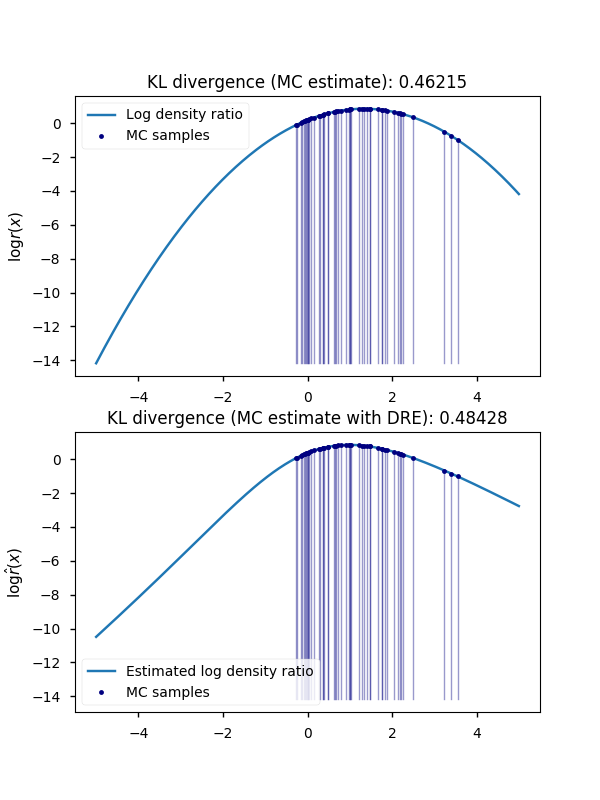##### Increasing MC sample size¶
In :
mc_samples = 5000

In :
samples_p = p.sample(sample_shape=(mc_samples,)).eval()

In :
# MC samples with analytical log density ratio
kl_mc = pd.Series(
log_density_ratio_gaussians(samples_p, mu_p, sigma_p, mu_q, sigma_q)
)

In :
# MC samples with density ratio estimator
kl_mc_dre = pd.Series(log_ratio_estimator.predict(samples_p).ravel())

In :
kl_estimates = pd.concat([kl_mc, kl_mc_dre], axis=1,
keys=['kl_mc', 'kl_mc_dre'])
kl_estimates.describe()

Out:
kl_mc kl_mc_dre
count 5000.000000 5000.000000
mean 0.437779 0.437814
std 0.596221 0.580184
min -5.995524 -5.625906
25% 0.301282 0.291181
50% 0.669817 0.655026
75% 0.816464 0.807633
max 0.859814 0.851987
In :
fig, ax = plt.subplots(figsize=golden_size(6))

sns.distplot(kl_estimates.kl_mc, ax=ax, label='Exact log density ratio')
sns.distplot(kl_estimates.kl_mc_dre, ax=ax,
label='Estimated log density ratio')

ax.axvline(x=kl_analytical, color='r', linewidth=2.,
label='KL Analytical')

ax.set_xlim(-1, 1)
ax.set_xlabel('log density ratio')

ax.legend()

plt.show()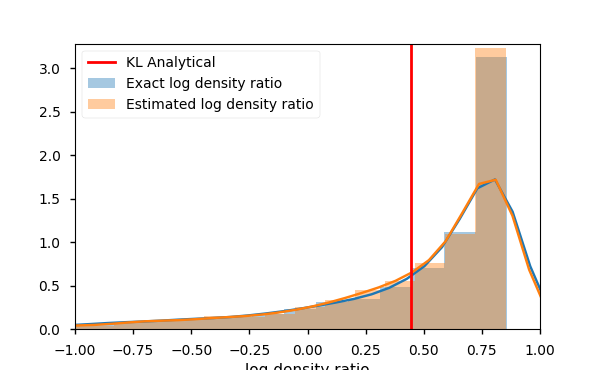In :
fig, ax = plt.subplots(figsize=golden_size(6))

sns.boxplot(x='estimator', y='sample',
data=pd.melt(kl_estimates,
var_name='estimator',
value_name='sample'), ax=ax)

ax.axhline(y=kl_analytical, color='r', linewidth=2.,
label='Analytical')

ax.set_ylim(-1, 1)

plt.show()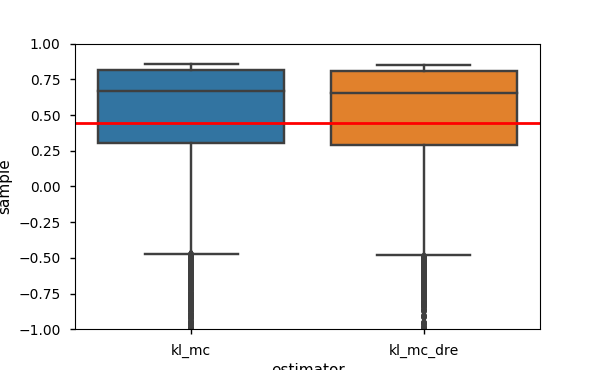In :
# Cumulative mean of MC samples
kl_estimates_cum_mean = kl_estimates.expanding().mean()

In :
fig, ax = plt.subplots(figsize=golden_size(6))

kl_estimates_cum_mean.plot(ax=ax, y='kl_mc',
label='MC Estimate (Exact)')

kl_estimates_cum_mean.plot(ax=ax, y='kl_mc_dre',
label='MC Estimate (DRE)', )

ax.axhline(y=kl_analytical, color='r', linewidth=2.,
label='Analytical')

ax.set_xlabel('Monte Carlo samples')
ax.set_ylabel('KL Divergence')

ax.legend()

plt.show()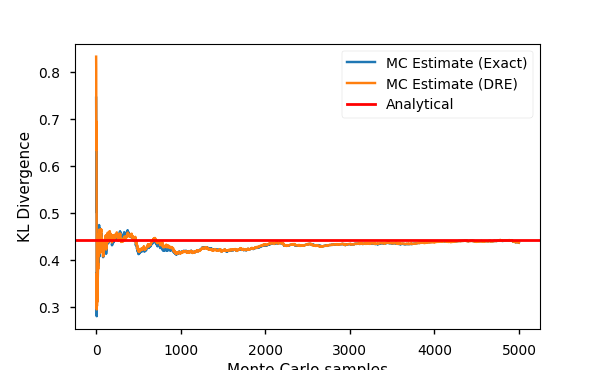##### Intermezzo: Symmetric KL (Jensen-Shannon) divergence¶

The Jensen-Shannon divergence is given by

$$\mathrm{JS}[p \| q] = \frac{1}{2}( \mathrm{KL}[p \| m] + \mathrm{KL}[q \| m] ),$$

where $m$ is the mixture density

$$m(x) = \frac{p(x) + q(x)}{2}.$$

This cannot be evaluated analytically (in closed-form) since the KL divergence between a Gaussian and a mixture of Gaussians is not available in closed-form. Hence, we estimate JS divergence by estimating its constituent KL divergence terms with Monte Carlo sampling.

In :
mc_samples = 10000

In :
x_p = p.sample(sample_shape=(mc_samples,)).eval()
x_q = q.sample(sample_shape=(mc_samples,)).eval()

In :
mixture_prob = lambda x, p, q: .5 * (p.prob(x) + q.prob(x))


$$\log \frac{p_1(x)}{(p_1(x) + p_2(x)) / 2} = \log p_1(x) - \log \{ p_1(x) + p_2(x) \} - \log 2$$

In :
def log_density_ratio_mixture(x, p1, p2):

return p1.log_prob(x) - tf.log(mixture_prob(x, p1, p2))

In :
def js_divergence_mc(x_p, x_q, p, q):

return .5 * (log_density_ratio_mixture(x_p, p, q) +
log_density_ratio_mixture(x_q, q, p))

In :
js_mc = pd.Series(js_divergence_mc(x_p, x_q, p, q).eval())
js_mc.mean()

Out:
0.12771526

Interestingly, the JS divergence is related to the binary cross-entropy loss,

\begin{align} \sup_{\theta} \left \{ \mathbb{E}_{p(x)} [\log D_{\theta}(x)] + \mathbb{E}_{q(x)} [\log(1-D_{\theta}(x))] \right\} & \leq 2 \cdot \mathrm{JS}[p \|q ] - \log 4 \\ & = 2 \left \{ \mathrm{JS}[p \|q ] - \log 2 \right \}. \end{align}

Negating both sides, we have

\begin{align} {-} \inf_{\theta} \left \{ {-} \mathbb{E}_{p(x)} [\log D_{\theta}(x)] {-} \mathbb{E}_{q(x)} [\log(1-D_{\theta}(x))] \right\} & \geq 2 \left \{ \log 2 - \mathrm{JS}[p \|q ] \right \}. \end{align}

Finally, halving both sides, we obtain $${-} \inf_{\theta} \left \{ - \frac{1}{2} \mathbb{E}_{p(x)} [\log D_{\theta}(x)] {-} \frac{1}{2} \mathbb{E}_{q(x)} [\log(1-D_{\theta}(x))] \right\} \geq \log 2 - \mathrm{JS}[p \|q ].$$

In :
np.log(2.) - js_mc.mean()

Out:
0.56543192076952475

Compare this with the last 10 values of the training and validation loss.

In :
hist_df.tail(n=10)

Out:
loss val_loss
240 0.574613 0.606388
241 0.574872 0.607644
242 0.574297 0.605857
243 0.574933 0.606334
244 0.575266 0.606556
245 0.574930 0.606873
246 0.575319 0.607448
247 0.574940 0.606458
248 0.575553 0.606798
249 0.574863 0.607222
In :
fig, ax = plt.subplots(figsize=golden_size(6))

hist_df = pd.DataFrame(hist.history)
hist_df.plot(ax=ax)

ax.axhline(y=np.log(2.) - js_mc.mean(),
color='r', linewidth=2.,
label='$\log 2 - \mathrm{JS}[p || q ]$')

ax.set_ylabel('loss')
ax.set_xlabel('epochs')

ax.legend()

plt.show()